• 网上找到的资源，代码比较基础，内有自带数据，可运行，大家可以下下来进行学习。
• 基于BP神经网络原理，针对数据当中所需的分类需求进行开发的小程序，具备一定的直到作用。
• 包含两个图像分类的资源 1.bp算法 用matlab实现 图像...2.基于神经网络的图像分类，数据资源是基于CIFAR-10，内含训练测试数据（batch），是唐宇迪深度学习入门课程的修改代码，python3版本 源码公开，仅供学习使用。
• 01-BP神经网络-分类,bp神经网络二分类,matlab源码.zip
• 题目： 企业到金融商业机构贷款，金融商业机构需要对企业进行评估...编写matlab代码，构造一个输入结点为2，中间隐藏层结点为5，输出结点为1的BP神经网络。其训练次数设置为200，训练目标最小误差设置为0.00001。用训练
题目： 企业到金融商业机构贷款，金融商业机构需要对企业进行评估。评估结果为0和1两种形式，0表示企业两年后破产，将拒绝贷款；而1表示企业2年后具备还款能力，可以贷款。表5-9中，已知前20家企业的三项评价指标值和评估结果，试建立神经网络，决策树模型对剩余5家企业进行评估。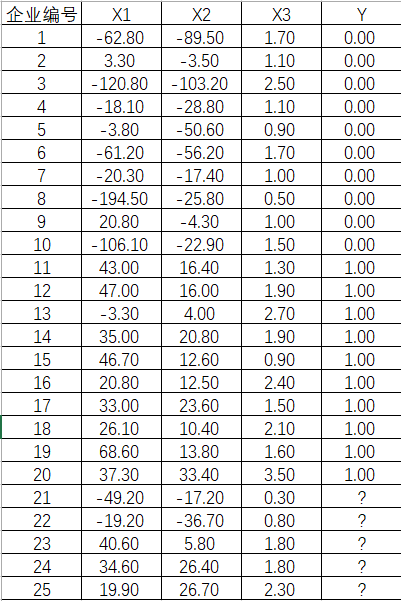(数据下载，无需积分)
解题过程：
建立双层前向神经网络模型：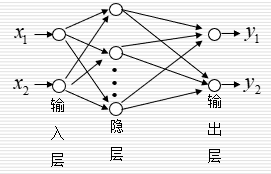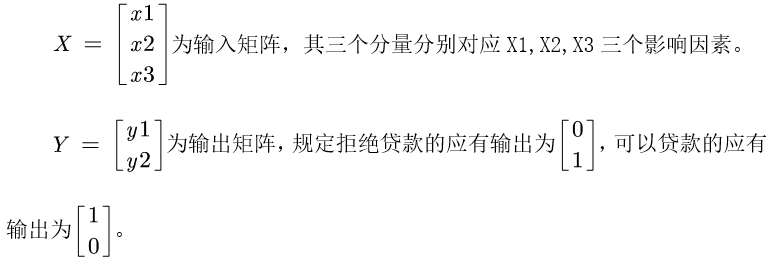编写matlab代码，构造一个输入结点为2，中间隐藏层结点为5，输出结点为1的BP神经网络。其训练次数设置为200，训练目标最小误差设置为0.00001。用训练样本对BP神经网络进行训练。训练效果如下：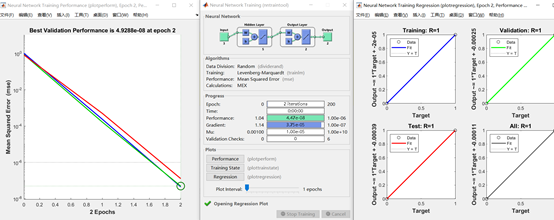检验样本的预测值（红线）和期望值（蓝线）如下所示：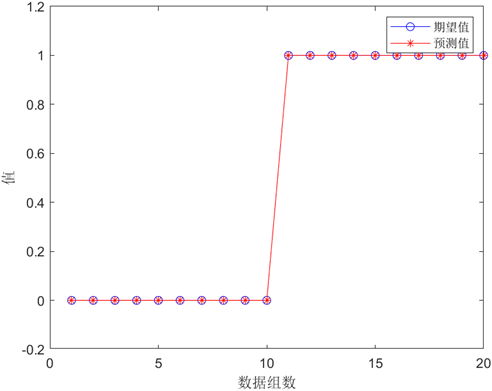将题目中未分类的五组数据作为预测集输入到我们构建的BP神经网络中，得到预测结果如下：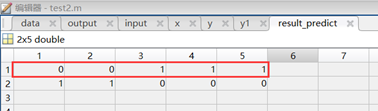因此，利用BP神经网络我们预测出以下结论：编号为21、22的企业银行将拒绝贷款，编号为23,24,25的企业银行可以贷款。
代码如下：
%% 清空环境变量
clear
clc
%% 读取数据
input = data(1:25,2:4)';%载入输入数据
output = data(1:20,5)'; %载入输出数据
x = input(:,1:20);
[xn,xps]=mapminmax(x);%归一化到[-1,1]之间
y = zeros(2,20);
for i=1:20
if output(1,i)==1
y(1,i)=1;
y(2,i)=0;
else
y(1,i)=0;
y(2,i)=1;
end
end
%% 建立两层前向神经网络
net=newff(xn,y,5,{'tansig','purelin'},'trainlm');
net.trainParam.goal=0.000001; %设定训练误差
net.trainParam.epochs=200;  %设定最大训练步数
net=train(net,x,y); %训练网络
y1=sim(net,x); %输出数据
%yy=sim(net,[1.24 1.28 1.40;1.80 1.84 2.04]-1)
%% 数据泛化
input_predict = input(:,21:25);
[input_predictn,input_predictps]=mapminmax(input_predict);%归一化到[-1,1]之间
result_predict = sim(net,input_predictn);
result_predict = round(result_predict);
%% 作图
figure(1)
yy = y(1,:);
y11 = y1(1,:);
plot(yy,'bo-')
hold on
plot(y11,'r*-')
% hold on
% plot(error,'square','MarkerFaceColor','b')
legend('期望值','预测值')
xlabel('数据组数')
ylabel('值')

展开全文数学建模 机器学习
• ## BP神经网络的MATLAB实现

万次阅读 多人点赞 2017-05-18 15:56:04
接下来，我们利用MATLABBP神经网络进行实现。我们直接上代码，并进行解释。MATLAB 代码整个代码是基于BP神经网络矩阵形式编写的，对公式有疑惑的同学可以参考下上篇博文。sigmoid.mfunction [ out ] = sigmoid( in...

算法流程
关于BP神经网络的公式推导，上一篇博文《BP神经网络原理简单介绍以及公式推导（矩阵形式和分量形式） 》已经做了详细的说明。接下来，我们利用MATLAB对BP神经网络进行实现。我们直接上代码，并进行解释。
MATLAB 代码
整个代码是基于BP神经网络矩阵形式编写的，对公式有疑惑的同学可以参考下上篇博文。
sigmoid.m
function [ out ] = sigmoid( in )
%SIGMOID Summary of this function goes here
%   Detailed explanation goes here

out = 1./(1+exp(-in));

end
。Sigmoid的函数一般形式有：

f(x)=11+e−2λx，f′(x)=2λf(x)(1−f(x))f(x)=1−e−2λx1−e−2λx,f′(x)=λ(1−f2(x))

f(x) = \frac{1}{1+e^{-2\lambda x}}， f'(x) = 2\lambda f(x)(1-f(x))\\
f(x) = \frac{1-e^{-2\lambda x}}{1-e^{-2\lambda x}}, f'(x) = \lambda(1-f^2(x))

它们导数都是自相关的。我们的激活函数就选择最简单的Sigmoid的函数，

f(x)=11+e−x
$f(x) = \frac{1}{1+e^{-x}}$，其导数为：

f′(x)=f(x)(1−f(x))
$f'(x) = f(x)(1-f(x))$

create_w.m
function [ W ] = create_w( n_levels )
%CREATE_W Summary of this function goes here
%   Detailed explanation goes here
n_level = numel(n_levels) - 1;
W = cell(n_level,1);

for i=1:n_level
W{i} = ones(n_levels(i+1), n_levels(i));
end

end
创建权值矩阵，初始值都是1。其中n_levels是一个向量，描述了神经网络的结构，比如
n_levels = [2 6 1]
描述了一个2-6-1的网络，也就是输入层2个神经元，隐藏层6个神经元，输出层1个神经元。上篇推导公式博文中的网络可以被描述为6-4-2-2网络。  每一层的权值矩阵与上一层的输入个数和这一层的输出个数有关。
create_theta.m
function [ theta ] = create_theta( levels )
%CREATE_THETA Summary of this function goes here
%   Detailed explanation goes here
n_level = numel(levels) - 1;
theta = cell(n_level, 1);

for i=1:n_level
theta{i} = ones(levels(i+1),1);
end

end
创建偏移量矩阵，初始值为1。
BP_predict.m
function [ output, Y ] = BP_predict( input, W, theta)
%BP_ Summary of this function goes here
%   Detailed explanation goes here
f = @sigmoid;
n_w = numel(W);
Y = cell(n_w+1, 1);
Y{1} = input';

for i=1:n_w
net = W{i}*Y{i} + theta{i};
Y{i+1} = f(net);
end
output = Y{end};
end
在训练中，需要对目前的

W
$W$和theta$theta$是否符合要求，因此需要进行预测。BP_predict是专门为了训练写的预测函数，只能对单个数据，返回值包括了中间层的输出

Y
$Y$。

BP_predict2.m

function [ output] = BP_predict2( input, W, theta)
%BP_ Summary of this function goes here
%   Detailed explanation goes here

f = @sigmoid;
n_w = numel(W);
y = input';

for i=1:n_w
net = bsxfun(@plus,W{i}*y,theta{i});
y = f(net);
end
output = y;
end

BP_predict2用于预测，可以对一个数据矩阵进行预测。

BP_tranning.m

function [ W,theta ] = BP_tranning( X, levels, step )
%BP_TRANNING Summary of this function goes here
%   Detailed explanation goes here
if nargin < 3
step = 0.001;
end

n_levels = size(levels, 2) - 1;
n_input = levels(1);
W = create_w(levels);
theta = create_theta(levels);

[n_data,col] = size(X);
n_label = col - n_input;

tranning_data = X(:,1:n_input); %Y0 is input
label = X(:,n_input+1:end)';
f = @sigmoid;

eps = 10e-7;
old_error = 0;
while true

for k=1:n_data
[output, Y] =  BP_predict(tranning_data(k,:), W, theta);
delta = label(:,k) - output;
% update the W, from the last layer to the first layer
for l=n_levels:-1:1
net = W{l}*Y{l} + theta{l};
if l == n_levels
S = diag(f(net).*(1-f(net)))*delta;
else
S = diag((f(net).*(1-f(net))))*W{l+1}'*S;
end
%dW = -S*Y{l}';
new_W{l} = W{l} + step*S*Y{l}';
new_theta{l} = theta{l} + step*S;
end % end update
W = new_W;
theta = new_theta;

end % end for

y = BP_predict2(tranning_data, W, theta);
delta = y - label;
error = sum(sum(delta.^2));
if abs(error - old_error) < eps;
break;
else
old_error = error;
end

end
end

训练的中止条件，个人觉得很难确定。不得已，写了一个不那么合理的：就是通过比较前后两次的错误率，错误率变化很小很小，说明错误率很难降低了，模型趋于稳定了。
其中Y{l}$Y\{l\}$，对应公式中的

Y(l−1)
$Y^{(l-1)}$，因为MATLAB中下标从1开始。
测试
demo_3class.m
%% Build a tranning set of three class
c_1 = [0 0];
c_2 = [0 1];
c_3 = [1 0];
c_4 = [1 1];

n_L1 = 20; % number of label 1
n_L2 = 20; % number of label 2
n_L3 = 20; % number of label 3

A = zeros(n_L1, 4);
A(:,3) = 0; A(:,4) = 0;
for i=1:n_L1
A(i,1:2) = c_1 + rand(1,2)/2;
end

B = zeros(n_L2, 4);
B(:,3) = 0; B(:,4) = 1;
for i=1:n_L2
B(i,1:2) = c_2 + rand(1,2)/2;
end
C = zeros(n_L3, 4);
C(:,3) = 1; C(:,4) = 0;
for i=1:n_L3
C(i,1:2) = c_3 + rand(1,2)/2;
end
D = zeros(n_L3, 4);
D(:,3) = 1; D(:,4) = 0;
for i=1:n_L3
D(i,1:2) = c_4 + rand(1,2)/2;
end
scatter(A(:,1), A(:,2),[],'r');
hold on
scatter(B(:,1), B(:,2),[],'g');
hold on
scatter(C(:,1), C(:,2),[],'k');
hold on
scatter(D(:,1), D(:,2),[],'k');
X = [A;B;C;D];

%% Trainning the BP network
dbstop if error
n_label = 2;
% create the weight matrix
n_input = size(X,2) - n_label;% number of feature of each data, here is 2, only x-axis and y-axis
levels = [n_input 7 n_label];

step = 0.1;

[W,theta] = BP_tranning(X, levels, step);
save three_class W theta

%% show the result
input = X(:,1:n_input);
label = X(:,n_input+1:end);
y = BP_predict2(input, W, theta);
Y = y';
Y(find(Y>0.5)) = 1;
Y(find(Y<=0.5)) = 0;
T = sum(label - Y, 2);
error_index = find(T ~= 0);
figure;
scatter(X(:,1), X(:,2), [], 'g');
hold on
scatter(X(error_index,1), X(error_index,2), [], 'r');

演示了一个三类分类器。对于多类分类，神经网络应该有多个输出，每个输出为0或者1，组合得到结果。比如这个例子，00表示第一类，01表示第二类，10表示第三类。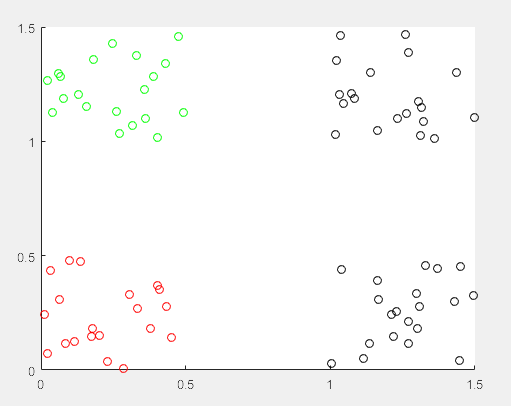上图是初始训练数据，每种颜色代表一类。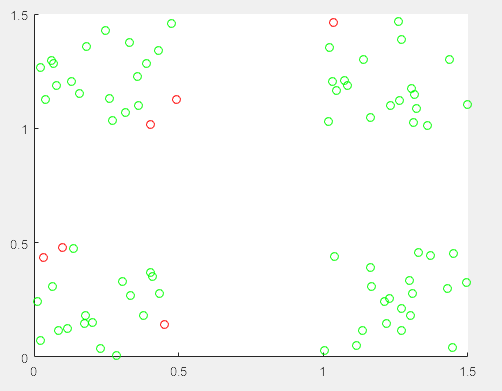上图是训练之后，对训练集进行预测的结果，其中红色表示错误，绿色表示正确。
总结
整体来说，实现BP算法不难，但是在实验部分却发现模型的选择、step的选择、终止条件的选择都很麻烦。已经隐约感受到调参民工的辛苦了。所有代码可以在这里下载
展开全文神经网络 反馈网络
• 在人工神经网络的实际应用中，BP网络广泛应用于函数逼近、模式识别/分类、数据压缩等，80%~90%的人工神经网络模型是采用BP网络或它的变化形式，它也是前馈网络的核心部分，体现了人工神经网络最精华的部分。
• 基于MATLABBP神经网络用于二分类，以蚊子分类为例。内有实验代码，实验运行结果。
• 本实验依托于教材《模式分类》第版第六章（公式符号与书中一致）实验内容：设计编写BP神经网络和RBF神经网络，对给定数据集进行分类测试，并将分类准确率与SVM进行对比。实验环境：matlab2016a数据集：数据集大小3...
本实验依托于教材《模式分类》第二版第六章（公式符号与书中一致）
实验内容：
设计编写BP神经网络和RBF神经网络，对给定数据集进行分类测试，并将分类准确率与SVM进行对比。
实验环境：
matlab2016a
数据集：
数据集大小3*3000，表示3000个样本，每个样本包含2个特征，第三行表示样本所属的分类。对于此次实验编写的BP神经网络和RBF神经网络，均将原始数据集分为训练集和测试集两部分，训练集含2700个样本，测试集300样本，并且采用10-折交叉验证，将数据集分为10份，每次将其中一份作为测试，剩余作为训练，总共进行10次验证，得到10个准确率，将10个准确率求平均作为最终的衡量指标，与SVM分类效果进行对比。
本实验数据集下载：sample_ex6.mat
BP网络和RBF网络相似但有所不同，因此分开阐述，先来设计BP网络。
BP神经网络：
BP网络有三层，输入层，隐含层，输出层，输入层与隐含层之间有权值Wji，隐含层与输出层之间有权值Wkj（i，j，k分别代表各层的神经元数目）。根据给定数据规模，可设计输入层3个神经元（
展开全文rbf神经网络 设计 测试
• ## matlabbp神经网络三分类

千次阅读 多人点赞 2019-03-17 10:39:54
笔者做了一个根据人的年龄判断人属于青少年、中年和老年人的一个bp神经网络分类。 %% 清空环境变量 clc clear %% 训练数据预测数据 data=xlsread('data.xlsx'); %从1到768间随机排序，产生随机数，防止过拟合 k=...
笔者做了一个根据人的年龄判断人属于青少年、中年和老年人的一个bp神经网络分类。
%% 清空环境变量 clc clear
%从1到768间随机排序，产生随机数，防止过拟合 k=rand(1,40);%产生由在(0, 1)之间均匀分布的随机数组成的数组。 [m,n]=sort(k);%m是排序好的向量，n 是 向量m中对k的索引,这样就将随机数的排列顺序变成整数序号了，即随机抽取的样本
input=data(:,1);%输入数据 group=data(:,2);%标签
%随机提取30个样本为训练样本，从训练集和测试集随机选出10个样本为预测样本 input_train=input(n(1:30),:)';%训练样本，要转置 output_train=group(n(1:30),:)';%训练标签，要转置 input_test=input(n(31:40),:)';%测试样本，要转置 output_test=group(n(31:40),:)';%测试标签，要转置
%输入数据归一化 [inputn,inputps]=mapminmax(input_train);
%% BP网络训练 % %初始化网络结构 net=newff(inputn,output_train,10);
net.trainParam.epochs=1000; net.trainParam.lr=0.1; net.trainParam.goal=0.0000004;
%% 网络训练 net=train(net,inputn,output_train);
%% BP网络预测 %预测数据归一化 inputn_test=mapminmax('apply',input_test,inputps);   %网络预测输出 BPoutput=sim(net,inputn_test);%预测标签
%% 结果分析 %根据网络输出找出数据属于哪类 BPoutput(find(BPoutput<1.5))=1; BPoutput(find(BPoutput>=1.5&BPoutput<2.5))=2; BPoutput(find(BPoutput>=2.5))=3; %% 结果分析 %画出预测种类和实际种类的分类图 figure(1) plot(BPoutput,'og') hold on plot(output_test,'r*'); legend('预测类别','输出类别') title('BP网络预测分类与实际类别比对','fontsize',12) ylabel('类别标签','fontsize',12) xlabel('样本数目','fontsize',12) ylim([-0.5 3.5])
%预测正确率 rightnumber=0; for i=1:size(output_test,2)     if BPoutput(i)==output_test(i)         rightnumber=rightnumber+1;     end end rightratio=rightnumber/size(output_test,2)*100;
sprintf('测试准确率=%0.2f',rightratio) 数据如下：第一列为人的年龄，第二列为标签。
91 训练集121  231  21  41  51  271  291  141  191 0-30为青少年342  372  392  422  462  472  552  582  652  432 31-65为中年933  663  703  693  723  773  793  893  943  1013 66以上为老年21 测试集1003  602  412  231  763  813  933  562  121
2  bp神经网络的训练函数名是train，所以输入的数据变量名不能写成train，以防两者冲突导致计算机无法辨别。可以写成train123.这样。
https://blog.csdn.net/weixin_42296976/article/details/81252809  帖子也对这个问题进行了阐述
3 bp神经网络训练函数newff等，及其参数参数调整，详解见：https://blog.csdn.net/ckzhb/article/details/60879570
4 想查看bp神经网络性能图、迭代图和预测线性回归图，详见操作：https://blog.csdn.net/Q1302182594/article/details/8790613
展开全文• 它是一种应用较为广泛的神经网络模型，多用于函数逼近、模型识别分类、数据压缩和时间序列预测等。BP网络由输入层、隐层和输出层组成，具有高度非线性和较强的泛化能力，但也存在收敛速度慢、迭...
• 在已知的神经网络基础上，通过北航出版社开源MATLAB神经网络开源训练和代码库来学习在MATLAB上建立和学习BP神经网络。 %% 该代码为基于BP网络的语言识别 %% 清空环境变量 %%clc %%clear %% 训练数据预测数据提取...
• 吴恩达网易云公开课《深度学习》week3--shallow_nn_model二分类
• 利用MATLAB 进行BP神经网络的预测（含有神经网络工具箱） 最近一段时间在研究如何利用预测其销量个数，在网上搜索了一下，发现了很多模型来预测，比如利用回归模型、时间序列模型，GM（1,1）模型，可是自己在结合...
• BP神经网络matlab代码实现 文章目录BP神经网络matlab代码实现1. BP神经网络的简介和结构参数1.1 BP神经网络的结构组成1.2 BP神经网络训练界面的参数解读2. 实现BP网络的步骤3. matlab代码编写4. BP代码运行结果4.1 ...机器学习 深度学习 网络
• BP神经网络进行模式识别 具体的BP神经网络详细说明请参考博客：https://www.jianshu.com/p/3d96dbf3f764 神经网络的基础编程可参考博客：https://www.cnblogs.com/heaad/archive/2011/03/07/1976443.html 例题详解 ...深度学习
• BP神经网络是反向传播网络（back propagation）的简称，它是一种多层前向网络，采用最小均方误差学习方式。 算法步骤： 代码如下： function example9_1() p = [1.78 1.96 1.86 1.72 2.00 2.00 1.96 1.74 1.64 1.82...算法
• 最近一段时间在研究如何利用...发现神经网络模型可以来预测，并且有很多是结合时间序列或者SVM（支持向量机）等组合模型来进行预测，本文结合实际数据，选取了常用的BP神经网络算法，其算法原理，因网上一大堆，所以...
• 一、实验内容 ...本次实验目的是用Bp神经网络对iris数据集进行分类，并进行分析。 、实验过程 1.将数据集加载到工作区：load iris.dat; 2.数据预处理 （1）将原始iris数据集随机抽取118行作为训练数...人工智能 模式识别
• 附加的 zip 文件包含实现两层神经网络所需的内容。 这有望成为更广泛的神经网络工具集合的第一部分。 它可用于训练和模拟具有两层（输入层和输出层）的 NN。 输出层的激活函数可以是简单的感知器规则，也可以是逻辑...
• 手动搭建了一个bp神经网路，对乳腺癌数据集进行分类。数据集从sklearn中获得，神经网络用numpy手动搭建。手动搭建了一个bp神经网路，对乳腺癌数据集进行分类。数据集从sklearn中获得，神经网络用numpy手动搭建。
• ## BP神经网络原理及matlab实例

万次阅读 多人点赞 2017-10-27 09:04:33
上一次我们讲了M-P模型，它实际上就是对单个神经元的一种建模，还不足以模拟人脑神经系统的功能。...BP网络就是一种简单的人工神经网络。  本文具体来介绍一下一种非常常见的神经网络模型——反
• 一、简介 1 概述\ BP(Back Propagation)神经网络是1986年由Rumelhart和McCelland为首的科研小组...BP神经网络是一种按误差逆传播算法训练的多层前馈网络，是目前应用最广泛的神经网络模型之一。BP网络能学习和存贮...网络 算法 机器学习 深度学习
• 这几天在各大媒体上接触到了人工智能机器学习，觉得很有意思，于是开始入门最简单的机器算法——神经网络训练算法（Neural Network Training）;以前一直觉得机器学习很高深，到处是超高等数学、线性代数、数理统计。...机器学习 人工智能 算法
• 本文主要内容包括： (1) 介绍神经网络基本原理 (2) Matlab实现前向神经网络的方法  第0节、引例   本文以Fisher的Iris数据集作为神经网络程序的测试数据集。Iris数据集可以在...
• 在上一篇博文中：Matlab实现BP神经网络和RBF神经网络（一） 中，我们讨论了BP网络设计部分，下面我们将设计RBF网络并将它们结果与SVM对比。数据格式不变，详情请看上一篇博文。RBF神经网络：RBF网络和BP网络都是非...设计 算法...

# bp神经网络二分类matlabmatlab 订阅